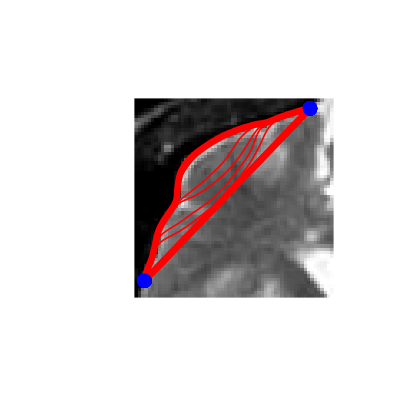# Active Contours using Parameteric Curves¶

This tour explores image segmentation using parametric active contours. $\newcommand{\dotp}{\langle #1, #2 \rangle}$ $\newcommand{\qandq}{\quad\text{and}\quad}$ $\newcommand{\qwhereq}{\quad\text{where}\quad}$ $\newcommand{\qifq}{ \quad \text{if} \quad }$ $\newcommand{\ZZ}{\mathbb{Z}}$ $\newcommand{\RR}{\mathbb{R}}$ $\newcommand{\CC}{\mathbb{C}}$ $\newcommand{\pa}{\left(#1\right)}$ $\newcommand{\si}{\sigma}$ $\newcommand{\Nn}{\mathcal{N}}$ $\newcommand{\Bb}{\mathcal{B}}$ $\newcommand{\EE}{\mathbb{E}}$ $\newcommand{\norm}{\|#1\|}$ $\newcommand{\abs}{\left|#1\right|}$ $\newcommand{\choice}{ \left\{ \begin{array}{l} #1 \end{array} \right. }$ $\newcommand{\al}{\alpha}$ $\newcommand{\la}{\lambda}$ $\newcommand{\ga}{\gamma}$ $\newcommand{\Ga}{\Gamma}$ $\newcommand{\La}{\Lambda}$ $\newcommand{\si}{\sigma}$ $\newcommand{\Si}{\Sigma}$ $\newcommand{\be}{\beta}$ $\newcommand{\de}{\delta}$ $\newcommand{\De}{\Delta}$ $\newcommand{\phi}{\varphi}$ $\newcommand{\th}{\theta}$ $\newcommand{\om}{\omega}$ $\newcommand{\Om}{\Omega}$

In :
options(warn=-1) # turns off warnings, to turn on: "options(warn=0)"

library(imager)
library(png)

for (f in list.files(path="nt_toolbox/toolbox_general/", pattern="*.R")) {
source(paste("nt_toolbox/toolbox_general/", f, sep=""))
}

for (f in list.files(path="nt_toolbox/toolbox_signal/", pattern="*.R")) {
source(paste("nt_toolbox/toolbox_signal/", f, sep=""))
}

source("nt_toolbox/toolbox_wavelet_meshes/meshgrid.R")
options(repr.plot.width=3.5, repr.plot.height=3.5)


## Parameteric Curves¶

In this tours, the active contours are represented using parametric curve $\ga : [0,1] \rightarrow \RR^2$.

This curve is discretized using a piewise linear curve with $p$ segments, and is stored as a complex vector of points in the plane $\ga \in \CC^p$.

Initial polygon.

In :
gamma0 <- c(.78, .14, .42, .18, .32, .16, .75, .83, .57, .68, .46, .40, .72, .79, .91, .90) + 1i*c(.87, .82, .75, .63, .34, .17, .08, .46, .50, .25, .27, .57, .73, .57, .75, .79)


Display the initial curve.

In :
periodize <- function(gamma){ c(gamma, gamma) }

cplot <- function(gamma, type, pch, lty, col, lw=2, add=FALSE){
plot(Re(periodize(gamma)), Im(periodize(gamma)), type=type, pch=pch, lty=lty, col=col, lwd=lw, xaxt='n', yaxt='n', ann=FALSE, bty="n")
}
else{
lines(Re(periodize(gamma)), Im(periodize(gamma)), type=type, pch=pch, lty=lty, col=col, lwd=lw, xaxt='n', yaxt='n', ann=FALSE, bty="n")
}

}

options(repr.plot.width=3.5, repr.plot.height=4.5)

cplot(gamma0, type="o", pch=1, lty=1, col="blue")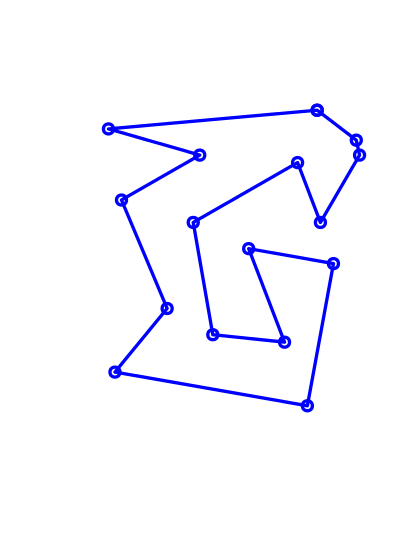Number of points of the discrete curve.

In :
p <- 256


Shortcut to re-sample a curve according to arc length.

In :
interpc <- function(x, xf, yf){ approx(xf, Re(yf), x)$y + 1i*approx(xf, Im(yf), x)$y }
curvabs <- function(gamma){ c(0, cumsum(1e-5 + abs(gamma[1:(length(gamma)-1)]-gamma[2:length(gamma)]))) }
resample1 <- function(gamma, d){ interpc((1:p)/p, d/d[length(d)], gamma)}
resample <- function(gamma){ resample1( periodize(gamma), curvabs(periodize(gamma)) ) }


Initial curve $\ga_1(t)$.

In :
gamma1 <- resample(gamma0)


Display the initial curve.

In :
cplot(gamma1, type="l", pch=1, lty=1, col="black")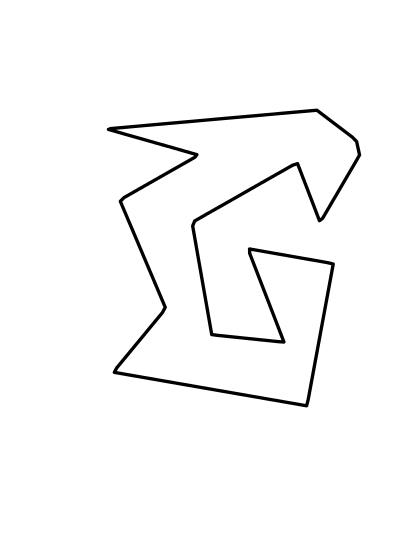Shortcut for forward and backward finite differences.

In :
shiftR <- function(c){ c(c[length(c)],c[1:(length(c)-1)]) }
shiftL <- function(c){ c(c[2:length(c)],c) }
BwdDiff <- function(c){ c - shiftR(c) }
FwdDiff <- function(c){ shiftL(c) - c }


The tangent to the curve is computed as $$t_\ga(s) = \frac{\ga'(t)}{\norm{\ga'(t)}}$$ and the normal is $n_\ga(t) = t_\ga(t)^\bot.$

Shortcut to compute the tangent and the normal to a curve.

In :
normalize <- function(v){ return( v/pmax( abs(v), 1e-10 ) ) }
tangent <- function(gamma) { normalize( FwdDiff(gamma) ) }
normal <- function(gamma){ -1i*tangent(gamma) }


Move the curve in the normal direction, by computing $\ga_1(t) \pm \delta n_{\ga_1}(t)$.

In :
delta <- .03
gamma2 <- gamma1 + delta * normal(gamma1)
gamma3 <- gamma1 - delta * normal(gamma1)


Display the curves.

In :
cplot(gamma1, type="l", pch=1, lty=1, col="black")
cplot(gamma2, type="l", pch=1, lty=2, col="red", add=TRUE)
cplot(gamma3, type="l", pch=1, lty=2, col="blue", add=TRUE)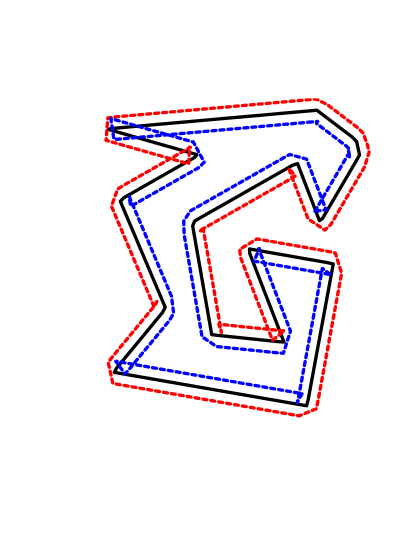## Evolution by Mean Curvature¶

A curve evolution is a series of curves $s \mapsto \ga_s$ indexed by an evolution parameter $s \geq 0$. The intial curve $\ga_0$ for $s=0$ is evolved, usually by minizing some energy $E(\ga)$ in a gradient descent $$\frac{\partial \ga_s}{\partial s} = \nabla E(\ga_s).$$

Note that the gradient of an energy is defined with respect to the curve-dependent inner product $$\dotp{a}{b} = \int_0^1 \dotp{a(t)}{b(t)} \norm{\ga'(t)} d t.$$ The set of curves can thus be thought as being a Riemannian surface.

The simplest evolution is the mean curvature evolution. It corresponds to minimization of the curve length $$E(\ga) = \int_0^1 \norm{\ga'(t)} d t$$

The gradient of the length is $$\nabla E(\ga)(t) = -\kappa_\ga(t) n_\ga(t)$$ where $\kappa_\ga$ is the curvature, defined as $$\kappa_\ga(t) = \frac{1}{\norm{\ga'(t)}} \dotp{ t_\ga'(t) }{ n_\ga(t) } .$$

Shortcut for normal times curvature $\kappa_\ga(t) n_\ga(t)$.

In :
normalC <- function(gamma){ BwdDiff(tangent(gamma)) / abs( FwdDiff(gamma) ) }


Time step for the evolution. It should be very small because we use an explicit time stepping and the curve has strong curvature.

In :
dt <- 0.001 / 100


Number of iterations.

In :
Tmax <- 3.0 / 100
niter <- round(Tmax/dt)


Initialize the curve for $s=0$.

In :
gamma <- gamma1


Evolution of the curve.

In :
gamma <- gamma + dt * normalC(gamma)


To stabilize the evolution, it is important to re-sample the curve so that it is unit-speed parametrized. You do not need to do it every time step though (to speed up).

In :
gamma <- resample(gamma)


Exercise 1: Perform the curve evolution. You need to resample it a few times.

In :
source("nt_solutions/segmentation_2_snakes_param/exo1.R")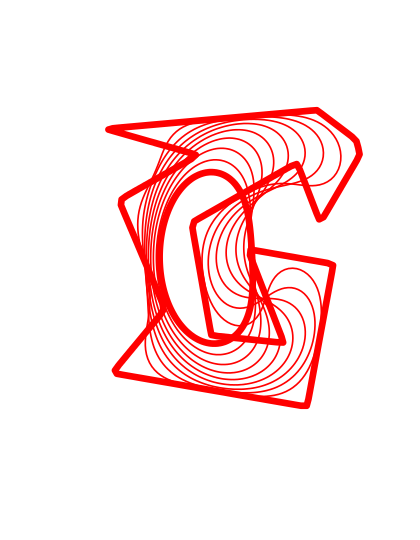## Geodesic Active Contours¶

Geodesic active contours minimize a weighted length $$E(\ga) = \int_0^1 W(\ga(t)) \norm{\ga'(t)} d t,$$ where $W(x)>0$ is the geodesic metric, that should be small in areas where the image should be segmented.

Size of the image $n$.

In :
n <- 200


Create a synthetic weight $W(x)$.

In :
nbumps <- 40
theta <- runif(nbumps)*2*pi
r <- .6*n/2
a <- c(.62*n,.6*n)
x <- round(a + r*cos(theta))
y <- round(a + r*sin(theta))
W <- matrix(rep(0, n*n), c(n,n))
for (i in 1:nbumps){
W[x[i], y[i]] <- 1
}
W <- gaussian_blur(W,6.0)
W <- rescale( -apply(W, c(1,2), min, 0.05), .3,1)


Display the metric $W$.

In :
options(repr.plot.width=3.5, repr.plot.height=3.5)

imageplot(W)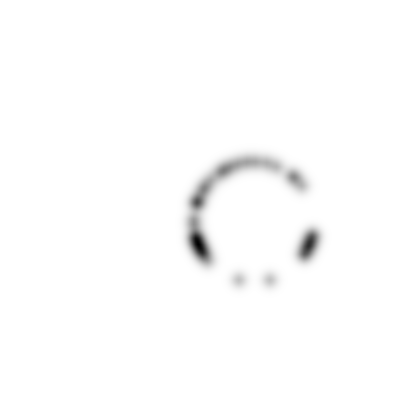Pre-compute the gradient $\nabla W(x)$ of the metric.

In :
G <- grad(W)
G <- G[,,1] + 1i*G[,,2]


Display the image of the magnitude $\norm{\nabla W(x)}$ of the gradient.

In :
imageplot(abs(G))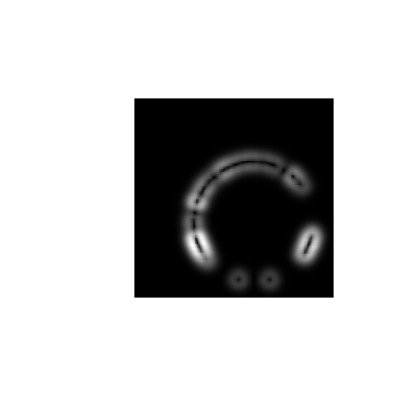Shortcut to evaluate the gradient and the potential along a curve.

In :
EvalG <- function(gamma){ bilinear_interpolate(G, Im(gamma), Re(gamma)) }
EvalW <- function(gamma){ bilinear_interpolate(W, Im(gamma), Re(gamma)) }


Create a circular curve $\ga_0$.

In :
r <- .98*n/2 # radius
p <- 128 # number of points on the curve
theta <- t( seq(0,2*pi,length=p+1) )
theta <- theta[1:length(theta)-1]
gamma0 <- n/2 * (1 + 1i) +  r*(cos(theta) + 1i*sin(theta))


Initialize the curve at time $t=0$ with a circle.

In :
gamma <- gamma0


For this experiment, the time step should be larger, because the curve is in $[0,n-1] \times [0,n-1]$.

In :
dt <- 1


Number of iterations.

In :
Tmax <- 5000
niter <- round(Tmax/dt)


Display the curve on the background.

In :
lw <- 2
imageplot(t(W))
cplot(gamma, type="l", pch=1, lty=1, col="red", add=TRUE)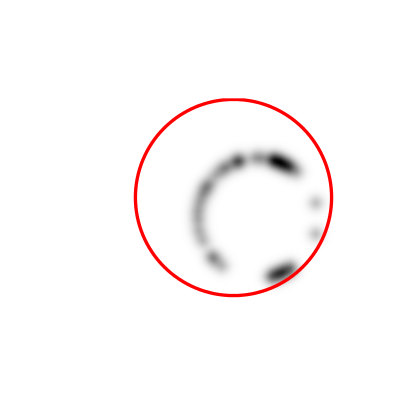The gradient of the energy is $$\nabla E(\ga) = -W(\ga(t)) \kappa_\ga(t) n_\ga(t) + \dotp{\nabla W(\ga(t))}{ n_\ga(t) } n_\ga(t).$$

Pointwise innerproduct on the curve.

In :
dotp <- function(c1,c2){ Re(c1)*Re(c2) + Im(c1)*Im(c2) }


Evolution of the curve according to this gradient.

In :
N <- normal(gamma)
g <- - EvalW(gamma) * normalC(gamma) + dotp(EvalG(gamma), N) * N
gamma <- gamma - dt*g


To avoid the curve from being poorly sampled, it is important to re-sample it evenly.

In :
gamma <- resample(gamma)


Exercise 2: Perform the curve evolution.

In :
source("nt_solutions/segmentation_2_snakes_param/exo2.R")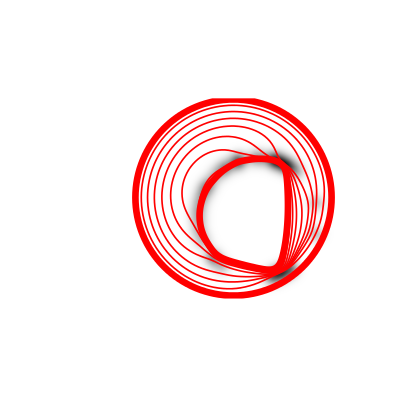# Medical Image Segmentation¶

One can use a gradient-based metric to perform edge detection in medical images.

Load an image $f$.

In :
n <- 256
name <- 'nt_toolbox/data/cortex.png'


Display.

In :
imageplot(f)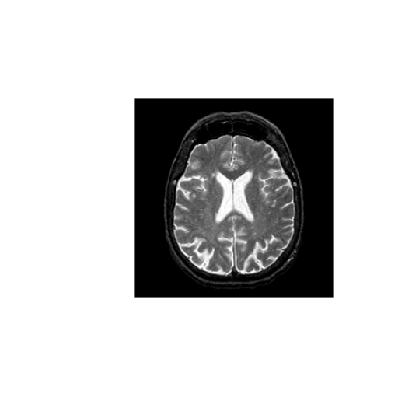An edge detector metric can be defined as a decreasing function of the gradient magnitude. $$W(x) = \psi( d \star h_a(x) ) \qwhereq d(x) = \norm{\nabla f(x)}.$$ where $h_a$ is a blurring kernel of width $a>0$.

Compute the magnitude of the gradient.

In :
G <- grad(f)
d0 <- sqrt(apply(G**2, c(1,2), sum))
imageplot(d0)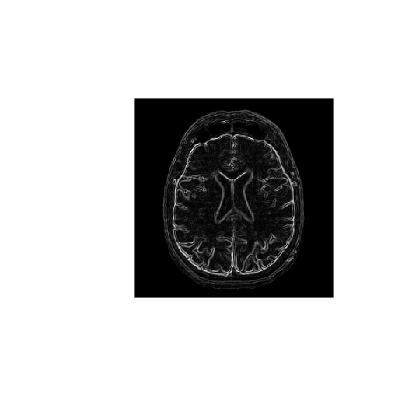Blur it by $h_a$.

In :
a <- 2
d <- gaussian_blur(d0, a)
imageplot(d)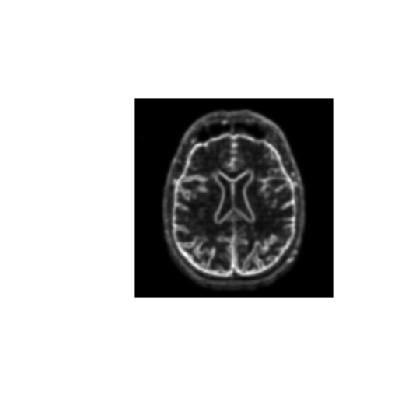Compute a decreasing function of the gradient to define $W$.

In :
d <- pmin(d, 0.4)
W <- rescale(-d, .8, 1)


Display it.

In :
imageplot(W)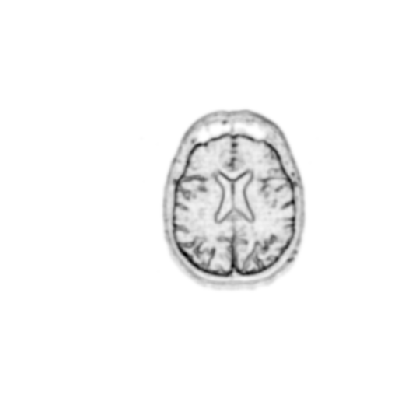Number of points.

In :
p <- 128


Exercise 3: Create an initial circle $\gamma_0$ of $p$ points. When plotting the image, you need to transpose it to have axis coherent with the cplot.

In :
source("nt_solutions/segmentation_2_snakes_param/exo3.R")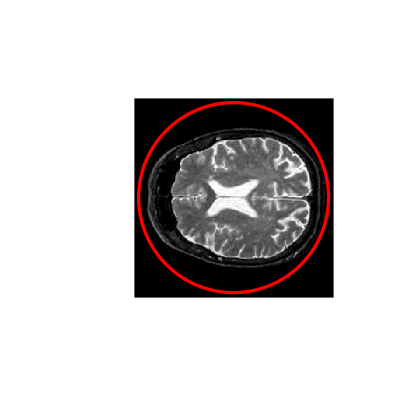Step size.

In :
dt <- 2


Number of iterations.

In :
Tmax <- 9000
niter <- round(Tmax/ dt)


Exercise 4: Perform the curve evolution.

In :
source("nt_solutions/segmentation_2_snakes_param/exo4.R")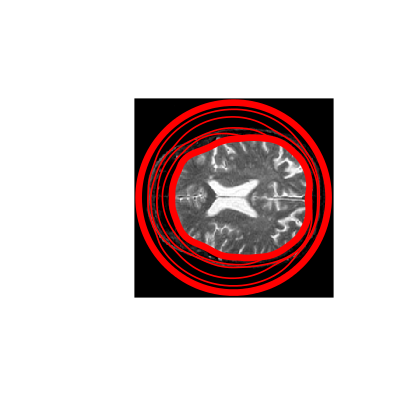# Evolution of a Non-closed Curve¶

It is possible to perform the evolution of a non-closed curve by adding boundary constraint $$\ga(0)=x_0 \qandq \ga(1)=x_1.$$

In this case, the algorithm find a local minimizer of the geodesic distance between the two points.

Note that a much more efficient way to solve this problem is to use the Fast Marching algorithm to find the global minimizer of the geodesic length.

Load an image $f$.

In :
n <- 256
f <- f[46:105, 61:120]
n <- dim(f)


Display.

In :
imageplot(f)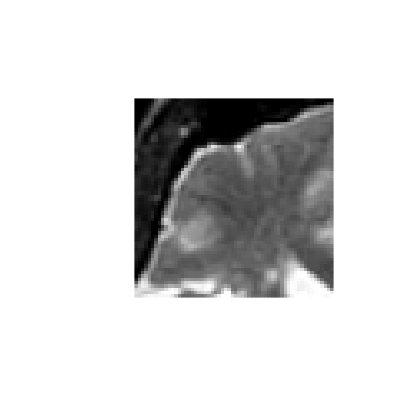Exercise 5: Compute an edge attracting criterion $W(x)>0$, that is small in area of strong gradient.

In :
source("nt_solutions/segmentation_2_snakes_param/exo5.R")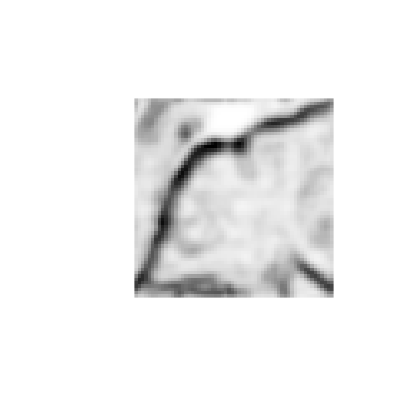Start and end points $x_0$ and $x_1$.

In :
x0 <- 4 + 55i
x1 <- 53 + 4i


Initial curve $\ga_0$.

In :
p <- 128
t <- t(seq(0, 1, length=p))
gamma0 <- t*x1 + (1-t)*x0


Initialize the evolution.

In :
gamma <- gamma0


Display.

In :
imageplot(t(W))
cplot(gamma, type="l", pch=1, lw=2, lty=1, col="red", add=TRUE)
points(Re(gamma), Im(gamma), pch=16, cex=1.5, col="blue")
points(Re(gamma[length(gamma)]), Im(gamma[length(gamma)]), pch=16, cex=1.5, col="blue")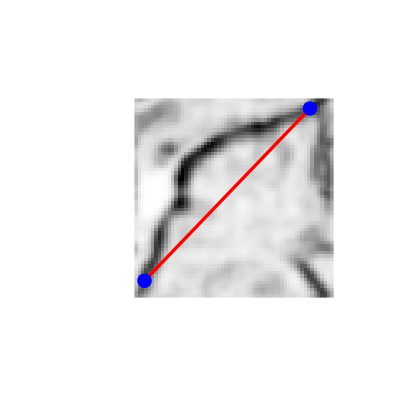Re-sampling for non-periodic curves.

In :
curvabs <- function(gamma){ c(0, cumsum(1e-5 + abs(gamma[1:(length(gamma)-1)]-gamma[2:length(gamma)]))) }
resample1 <- function(gamma, d){ interpc((1:p)/p, d/d[length(d)], gamma)}
resample <- function(gamma){ resample1( gamma, curvabs(gamma) ) }


Time step.

In :
dt <- 1/10


Number of iterations.

In :
Tmax <- 2000*4/ 7
niter <- round(Tmax/ dt)


Exercise 6: Perform the curve evolution. Be careful to impose the boundary conditions at each step.

In :
source("nt_solutions/segmentation_2_snakes_param/exo6.R")